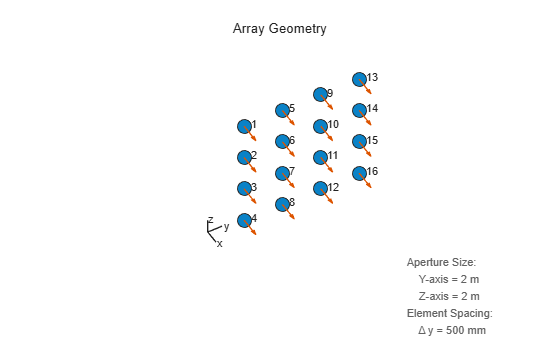# phased.URA

Uniform rectangular array

## Description

To create a Uniform Rectangular Array (URA) System object™:

1. Create the `phased.URA` object and set its properties.

2. Call the object with arguments, as if it were a function.

## Creation

### Syntax

``array = phased.URA``
``array = phased.URA(Name,Value)``
``array = phased.URA(SZ,D,Name,Value)``

### Description

````array = phased.URA` creates a uniform rectangular `array` (URA) System object that models a URA formed from identical isotropic phased array elements. Array elements are contained in the yz-plane in a rectangular lattice. The array look direction (boresight) points along the positive x-axis.```
````array = phased.URA(Name,Value)` creates an `array` object with each specified property Name set to the specified Value. You can specify additional name-value pair arguments in any order as (`Name1`,`Value1`, ..., `NameN`,`ValueN`). All properties needed to fully specify this object can be found in Properties.Response of 2-by-2 URA of Short-Dipole Antennas```
````array = phased.URA(SZ,D,Name,Value)` creates a `phased.URA` `array` System object with its `Size` property set to `SZ` and its `ElementSpacing` property set to `D`. Other specified property Names are set to the specified Values. `SZ` and `D` are value-only arguments. When specifying a value-only argument, specify all preceding value-only arguments. You can specify name-value pair arguments in any order.```

## Properties

expand all

Unless otherwise indicated, properties are nontunable, which means you cannot change their values after calling the object. Objects lock when you call them, and the `release` function unlocks them.

If a property is tunable, you can change its value at any time.

Phased array element, specified as a Phased Array System Toolbox antenna, microphone, or transducer element or Antenna Toolbox antenna.

Example: `phased.CosineAntennaElement`

Array size, specified as a 1-by-2 vector of integers or a single integer. containing the size of the array. If `Size` is a 1-by-2 vector, the vector has the form `[NumberOfRows, NumberOfColumns]`. If `Size` is a scalar, the array has the same number of elements in each row and column. For a URA, array elements are indexed from top to bottom along a column and continuing to the next columns from left to right. In this illustration, a `Size` value of `[3,2]` array has three rows and two columns.Example: `[3,2]`

Data Types: `double`

Element spacing, specified as a positive scalar or 1-by-2 vector of positive values. If `ElementSpacing` is a 1-by-2 vector, it has the form `[SpacingBetweenRows,SpacingBetweenColumns]`. See Spacing Between Columns and Spacing Between Rows. If `ElementSpacing` is a scalar, both row and column spacings are equal. Units are in meters.

Example: `[0.3, 0.5]`

Data Types: `double`

Element lattice type, specified as `'Rectangular'` or `'Triangular'`. When you set the `Lattice` property to `'Rectangular'`, all elements of the URA are aligned in both row and column directions. When you set the `Lattice` property to `'Triangular'`, elements in even rows are displaced toward the positive row axis direction. The displacement is one-half the element spacing along the row.

Example: `'Triangular'`

Data Types: `char` | `string`

Array normal direction, specified as one of `'x'`, `'y'`, or `'z'`. URA elements lie in a plane orthogonal to the selected array normal direction. Element boresight directions point along the array normal direction.

ArrayNormal Property ValueElement Positions and Boresight Directions
`'x'`Array elements lie on the yz-plane. All element boresight vectors point along the x-axis. This is the default value.
`'y'`Array elements lie on the zx-plane. All element boresight vectors point along the y-axis.
`'z'`Array elements lie on the xy-plane. All element boresight vectors point along the z-axis.

Element tapers, specified as a complex-valued scalar, complex-valued 1-by-MN vector, or complex-valued M-by-N matrix. Tapers are applied to each element in the sensor array. Tapers are often referred to as element weights. M is the number of elements along the z-axis, and N is the number of elements along y-axis. M and N correspond to the values of `[NumberofRows,NumberOfColumns]` in the `SIze` property. If `Taper` is a scalar, the same taper value is applied to all elements. If the value of `Taper` is a vector or matrix, taper values are applied to the corresponding elements. Tapers are used to modify both the amplitude and phase of the received data.

Example: `[0.4 1 0.4]`

Data Types: `double`

## Usage

### Syntax

``RESP = array(FREQ,ANG)``

### Description

example

````RESP = array(FREQ,ANG)` returns the responses of the array elements, `RESP`, at the operating frequencies specified in `FREQ` and directions specified in `ANG`. NoteThe object performs an initialization the first time the object is executed. This initialization locks nontunable properties and input specifications, such as dimensions, complexity, and data type of the input data. If you change a nontunable property or an input specification, the System object issues an error. To change nontunable properties or inputs, you must first call the `release` method to unlock the object. ```

### Input Arguments

expand all

Array operating frequencies, specified as a length-L row vector. Typical values are within the range specified by a frequency range property of the array element. That property is called `FrequencyRange` or `FrequencyVector`, depending on the type of array element. The element has zero response at frequencies outside that range. Units are in Hz.

Data Types: `double`

Array response directions, specified as a 2-by-M matrix or length-M row vector.

If `ANG` is a 2-by-M matrix, each column of the matrix specifies the direction in the form ```[azimuth; elevation]```. The azimuth angle must lie between –180° and 180°, inclusive. The elevation angle must lie between –90° and 90°, inclusive.

If `ang` is a row vector of length M, each element specifies the azimuth angle of the direction. In this case, the corresponding elevation angle is assumed to be 0°.

Units are in degrees.

### Output Arguments

expand all

Voltage responses of phased array elements. The output depends on whether or not the array supports polarization.

• If the array is not capable of supporting polarization, the voltage response, `RESP`, has the dimensions N-by-M-by-L. N is the number of elements in the array. The dimension M is the number of angles specified in `ANG`. L is the number of frequencies specified in `FREQ`. For any element, the columns of `RESP` contain the responses of the array elements for the corresponding direction specified in `ang`. Each of the L pages of `RESP` contains the responses of the array elements for the corresponding frequency specified in `freq`.

• If the array is capable of supporting polarization, the voltage response `RESP` is a MATLAB `struct` containing two fields, `RESP.H` and `RESP.V`. The field, `RESP.H`, represents the array’s horizontal polarization response, while `RESP.V` represents the array’s vertical polarization response. Each field has the dimensions N-by-M-by-L. N is the number of elements in the array, and M is the number of angles specified in `ANG`. L is the number of frequencies specified in `FREQ`. Each column of `RESP` contains the responses of the array elements for the corresponding direction specified in `ANG`. Each of the L pages of `ANG` contains the responses of the array elements for the corresponding frequency specified in `FREQ`.

## Object Functions

To use an object function, specify the System object as the first input argument. For example, to release system resources of a System object named `obj`, use this syntax:

`release(obj)`

expand all

 `beamwidth` Compute and display beamwidth of an array `collectPlaneWave` Simulate received plane waves at array `directivity` Compute array directivity `getElementNormal` Normal vectors for array elements `getElementPosition` Positions of array elements `getNumElements` Number of elements in an array `getTaper` Array element tapers `isPolarizationCapable` Array polarization capability `pattern` Plot array directivity and patterns `patternAzimuth` Plot array directivity or pattern versus azimuth `patternElevation` Plot array directivity or pattern versus elevation `perturbations` Perturbations defined on array `perturbedArray` Apply perturbations to phased array `perturbedPattern` Display pattern of perturbed array `plotGratingLobeDiagram` Plot grating lobe diagram of array `viewArray` View array geometry
 `step` Run System object algorithm `release` Release resources and allow changes to System object property values and input characteristics `reset` Reset internal states of System object

## Examples

collapse all

Construct a 2-by-2 rectangular lattice URA of short-dipole antenna elements. Then, find the response of each element at boresight. Assume the operating frequency is 1 GHz.

```sSD = phased.ShortDipoleAntennaElement; sURA = phased.URA('Element',sSD,'Size',[2 2]); fc = 1e9; ang = [0;0]; resp = step(sURA,fc,ang); disp(resp.V)```
``` -1.2247 -1.2247 -1.2247 -1.2247 ```

Construct a 3-by-2 rectangular lattice URA. By default, the array consists of isotropic antenna elements. Find the response of each element at boresight, 0 degrees azimuth and elevation. Assume the operating frequency is 1 GHz.

```array = phased.URA('Size',[3 2]); fc = 1e9; ang = [0;0]; resp = array(fc,ang); disp(resp)```
``` 1 1 1 1 1 1 ```

Plot the azimuth pattern of the array.

```c = physconst('LightSpeed'); pattern(array,fc,[-180:180],0,'PropagationSpeed',c, ... 'CoordinateSystem','polar','Type','powerdb','Normalize',true)```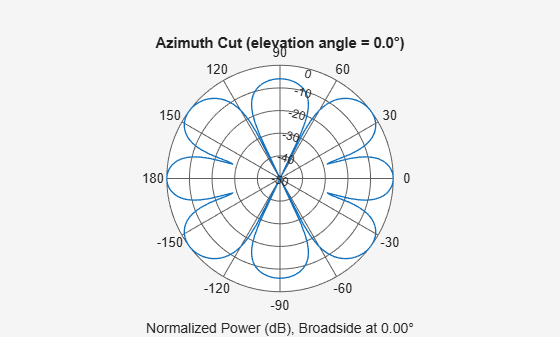This example shows how to find and plot the positions of the elements of a 5-row-by-6-column URA with a triangular lattice and a URA with a rectangular lattice. The element spacing is 0.5 meters for both lattices.

Create the arrays.

```h_tri = phased.URA('Size',[5 6],'Lattice','Triangular'); h_rec = phased.URA('Size',[5 6],'Lattice','Rectangular');```

Get the element y,z positions for each array. All the x coordinates are zero.

```pos_tri = getElementPosition(h_tri); pos_rec = getElementPosition(h_rec); pos_yz_tri = pos_tri(2:3,:); pos_yz_rec = pos_rec(2:3,:);```

Plot the element positions in the yz-plane.

```figure; gcf.Position = [100 100 300 400]; subplot(2,1,1); plot(pos_yz_tri(1,:), pos_yz_tri(2,:), '.') axis([-1.5 1.5 -2 2]) xlabel('y'); ylabel('z') title('Triangular Lattice') subplot(2,1,2); plot(pos_yz_rec(1,:), pos_yz_rec(2,:), '.') axis([-1.5 1.5 -2 2]) xlabel('y'); ylabel('z') title('Rectangular Lattice')```Construct a 5-by-2 element URA with a Taylor window taper along each column. The tapers form a 5-by-2 matrix.

```taper = taylorwin(5); ha = phased.URA([5,2],'Taper',[taper,taper]); w = getTaper(ha)```
```w = 10×1 0.5181 1.2029 1.5581 1.2029 0.5181 0.5181 1.2029 1.5581 1.2029 0.5181 ```

Simulate two received random signals at a 6-element URA. The array has a rectangular lattice with two elements in the row direction and three elements in the column direction. The signals arrive from 10° and 30° azimuth. Both signals have an elevation angle of 0°. Assume the propagation speed is the speed of light and the carrier frequency of the signal is 100 MHz.

```array = phased.URA([2 3]); fc = 100e6; y = collectPlaneWave(array,randn(4,2),[10 30],fc,physconst('LightSpeed'));```

Compute the directivity of two uniform rectangular arrays (URA). The first array consists of isotropic antenna elements. The second array consists of cosine antenna elements. In addition, compute the directivity of the first array steered to a specific direction.

Array of isotropic antenna elements

First, create a 10-by-10-element URA of isotropic antenna elements spaced one-quarter wavelength apart. Set the signal frequency to 800 MHz.

```c = physconst('LightSpeed'); fc = 3e8; lambda = c/fc; myAntIso = phased.IsotropicAntennaElement; myArray1 = phased.URA; myArray1.Element = myAntIso; myArray1.Size = [10,10]; myArray1.ElementSpacing = [lambda*0.25,lambda*0.25]; ang = [0;0]; d = directivity(myArray1,fc,ang,'PropagationSpeed',c)```
```d = 15.7753 ```

Array of cosine antenna elements

Next, create a 10-by-10-element URA of cosine antenna elements also spaced one-quarter wavelength apart.

```myAntCos = phased.CosineAntennaElement('CosinePower',[1.8,1.8]); myArray2 = phased.URA; myArray2.Element = myAntCos; myArray2.Size = [10,10]; myArray2.ElementSpacing = [lambda*0.25,lambda*0.25]; ang = [0;0]; d = directivity(myArray2,fc,ang,'PropagationSpeed',c)```
```d = 19.7295 ```

The directivity is increased due to the directivity of the cosine antenna elements.

Steered array of isotropic antenna elements

Finally, steer the isotropic antenna array to 30 degrees in azimuth and examine the directivity at the steered angle.

```ang = [30;0]; w = steervec(getElementPosition(myArray1)/lambda,ang); d = directivity(myArray1,fc,ang,'PropagationSpeed',c,... 'Weights',w)```
```d = 15.3309 ```

The directivity is maximum in the steered direction and equals the directivity of the unsteered array at boresight.

Construct three 2-by-2 URA's with element normals along the x-, y-, and z-axes. Obtain the element positions and normal directions.

First, choose the array normal along the x-axis.

```sURA1 = phased.URA('Size',[2,2],'ArrayNormal','x'); pos = getElementPosition(sURA1)```
```pos = 3×4 0 0 0 0 -0.2500 -0.2500 0.2500 0.2500 0.2500 -0.2500 0.2500 -0.2500 ```
`normvec = getElementNormal(sURA1)`
```normvec = 2×4 0 0 0 0 0 0 0 0 ```

All elements lie in the yz-plane and the element normal vectors point along the x-axis (0°,0°).

Next, choose the array normal along the y-axis.

```sURA2 = phased.URA('Size',[2,2],'ArrayNormal','y'); pos = getElementPosition(sURA2)```
```pos = 3×4 0.2500 0.2500 -0.2500 -0.2500 0 0 0 0 0.2500 -0.2500 0.2500 -0.2500 ```
`normvec = getElementNormal(sURA2)`
```normvec = 2×4 90 90 90 90 0 0 0 0 ```

All elements lie in the zx-plane and the element normal vectors point along the y-axis (90°,0°).

Finally, set the array normal along the z-axis. Obtain the normal vectors of the odd-numbered elements.

```sURA3 = phased.URA('Size',[2,2],'ArrayNormal','z'); pos = getElementPosition(sURA3)```
```pos = 3×4 -0.2500 -0.2500 0.2500 0.2500 0.2500 -0.2500 0.2500 -0.2500 0 0 0 0 ```
`normvec = getElementNormal(sURA3,[1,3])`
```normvec = 2×2 0 0 90 90 ```

All elements lie in the xy-plane and the element normal vectors point along the z-axis (0°,90°).

Construct a default URA with a rectangular lattice, and obtain the element positions.

```array = phased.URA; pos = getElementPosition(array)```
```pos = 3×4 0 0 0 0 -0.2500 -0.2500 0.2500 0.2500 0.2500 -0.2500 0.2500 -0.2500 ```

Construct a default URA, and obtain the number of elements.

```array = phased.URA; N = getNumElements(array)```
```N = 4 ```

Construct a 5-by-2 element URA with a Taylor window taper along each column. Then, draw the array showing the element taper shading.

```taper = taylorwin(5); array = phased.URA([5,2],'Taper',[taper,taper]); w = getTaper(array)```
```w = 10×1 0.5181 1.2029 1.5581 1.2029 0.5181 0.5181 1.2029 1.5581 1.2029 0.5181 ```
`viewArray(array,'ShowTaper',true)`Show that a URA array of `phased.ShortDipoleAntennaElement` short-dipole antenna elements supports polarization.

```antenna = phased.ShortDipoleAntennaElement('FrequencyRange',[1e9 10e9]); array = phased.URA([3,2],'Element',antenna); isPolarizationCapable(array)```
```ans = logical 1 ```

The returned value `1` shows that this array supports polarization.

Create a 5x7-element URA operating at 1 GHz. Assume the elements are spaced one-half wavelength apart. Show the 3-D array patterns.

Create the array

```sSD = phased.ShortDipoleAntennaElement(... 'FrequencyRange',[50e6,1000e6],... 'AxisDirection','Z'); fc = 500e6; c = physconst('LightSpeed'); lam = c/fc; sURA = phased.URA('Element',sSD,... 'Size',[5,7],... 'ElementSpacing',0.5*lam);```

Call the `step` method

Evaluate the fields of the first five elements at 45 degrees azimuth and 0 degrees elevation.

```ang = [45;0]; resp = step(sURA,fc,ang); disp(resp.V(1:5))```
``` -1.2247 -1.2247 -1.2247 -1.2247 -1.2247 ```

Display the 3-D directivity pattern at 1 GHz in polar coordinates

```pattern(sURA,fc,[-180:180],[-90:90],... 'CoordinateSystem','polar',... 'Type','directivity','PropagationSpeed',c)```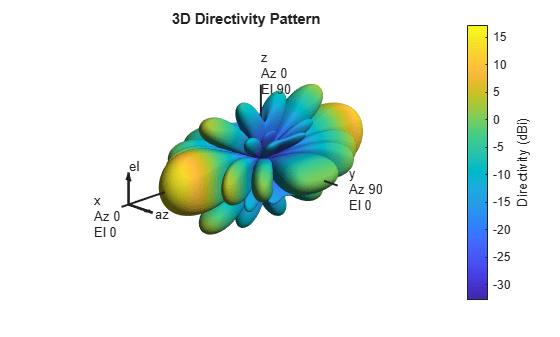Display the 3-D directivity pattern at 1 GHz in UV coordinates

```pattern(sURA,fc,[-1.0:.01:1.0],[-1.0:.01:1.0],... 'CoordinateSystem','uv',... 'Type','directivity','PropagationSpeed',c)```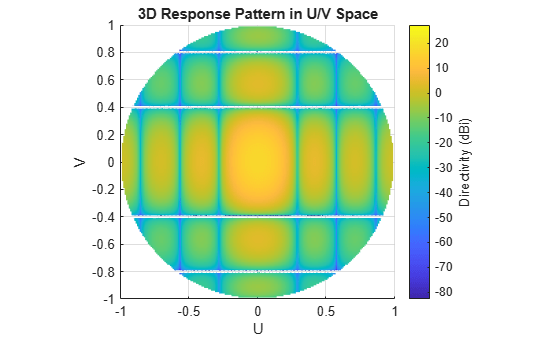Create a 5x7-element URA of short-dipole antenna elements operating at 1 GHz. Assume the elements are spaced one-half wavelength apart. Plot the array azimuth directivity patterns for two different elevation angles, 0 and 15 degrees. The `patternAzimuth` method always plots the array pattern in polar coordinates.

Create the array

```sSD = phased.ShortDipoleAntennaElement(... 'FrequencyRange',[50e6,1000e6],... 'AxisDirection','Z'); fc = 1e9; c = physconst('LightSpeed'); lam = c/fc; sURA = phased.URA('Element',sSD,... 'Size',[5,7],... 'ElementSpacing',0.5*lam);```

Display the pattern

Display the azimuth directivity pattern at 1 GHz in polar coordinates

```patternAzimuth(sURA,fc,[0 15],... 'PropagationSpeed',c,... 'Type','directivity')```Display a subset of angles

You can plot a smaller range of azimuth angles by setting the `Azimuth` parameter.

```patternAzimuth(sURA,fc,[0 15],... 'PropagationSpeed',c,... 'Type','directivity',... 'Azimuth',[-45:45])```Create a 7x7-element URA of backbaffled omnidirectional transducer elements operating at 2 kHz. Assume the speed of sound in water is 1500 m/s. The elements are spaced less than one-half wavelength apart. Plot the array elevation directivity patterns for three different azimuth angles, -20, 0, and 15 degrees. The `patternElevation` method always plots the array pattern in polar coordinates.

Create the array

```element = phased.OmnidirectionalMicrophoneElement(... 'FrequencyRange',[20,3000],... 'BackBaffled',true); fc = 1000; c = 1500; lam = c/fc; array = phased.URA('Element',element,... 'Size',[7,7],... 'ElementSpacing',0.45*lam);```

Display the pattern

Display the azimuth directivity pattern at 1 GHz in polar coordinates.

```patternElevation(array,fc,[-20, 0, 15],... 'PropagationSpeed',c,... 'Type','directivity')```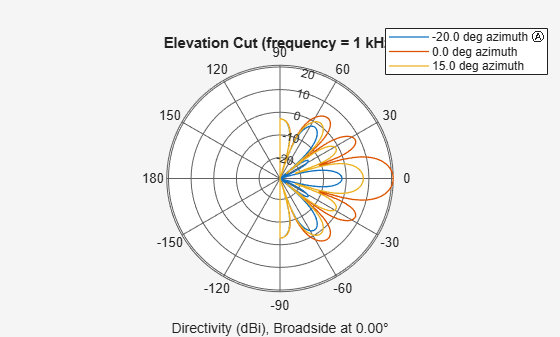Display a subset of elevation angles

You can plot a smaller range of elevation angles by setting the `Elevation` parameter.

```patternElevation(array,fc,[-20, 0, 15],... 'PropagationSpeed',c,... 'Type','directivity',... 'Elevation',[-45:45])```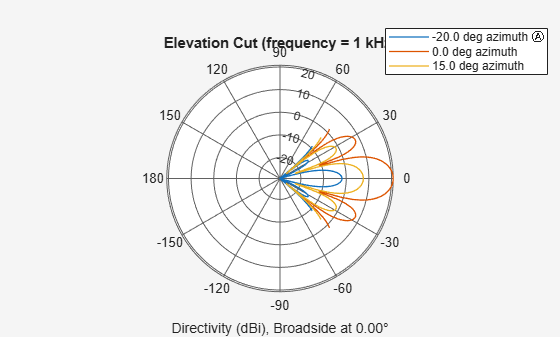Plot the grating lobe diagram for an 11-by-9-element uniform rectangular array having element spacing equal to one-half wavelength.

Assume the operating frequency of the array is 10 kHz. All elements are omnidirectional microphone elements. Steer the array in the direction 20 degrees in azimuth and 30 degrees in elevation. The speed of sound in air is 344.21 m/s at 21 deg C.

```cair = 344.21; f = 10.0e3; lambda = cair/f; microphone = phased.OmnidirectionalMicrophoneElement(... 'FrequencyRange',[20 20000]); array = phased.URA('Element',microphone,'Size',[11,9],... 'ElementSpacing',0.5*lambda*[1,1]); plotGratingLobeDiagram(array,f,[20;30],cair);```Plot the grating lobes. The main lobe of the array is indicated by a filled black circle. The grating lobes in visible and nonvisible regions are indicated by unfilled black circles. The visible region is the region in u-v coordinates for which ${\mathit{u}}^{2}+{\mathit{v}}^{2}\le 1$. The visible region is shown as a unit circle centered at the origin. Because the array spacing is less than one-half wavelength, there are no grating lobes in the visible region of space. There are an infinite number of grating lobes in the nonvisible regions, but only those in the range [-3,3] are shown.

The grating-lobe free region, shown in green, is the range of directions of the main lobe for which there are no grating lobes in the visible region. In this case, it coincides with the visible region.

The white areas of the diagram indicate a region where no grating lobes are possible.

This example shows how to display the element positions, normal directions, and indices for all elements of a 4-by-4 square URA.

```ha = phased.URA(4); viewArray(ha,'ShowNormals',true,'ShowIndex','All');```